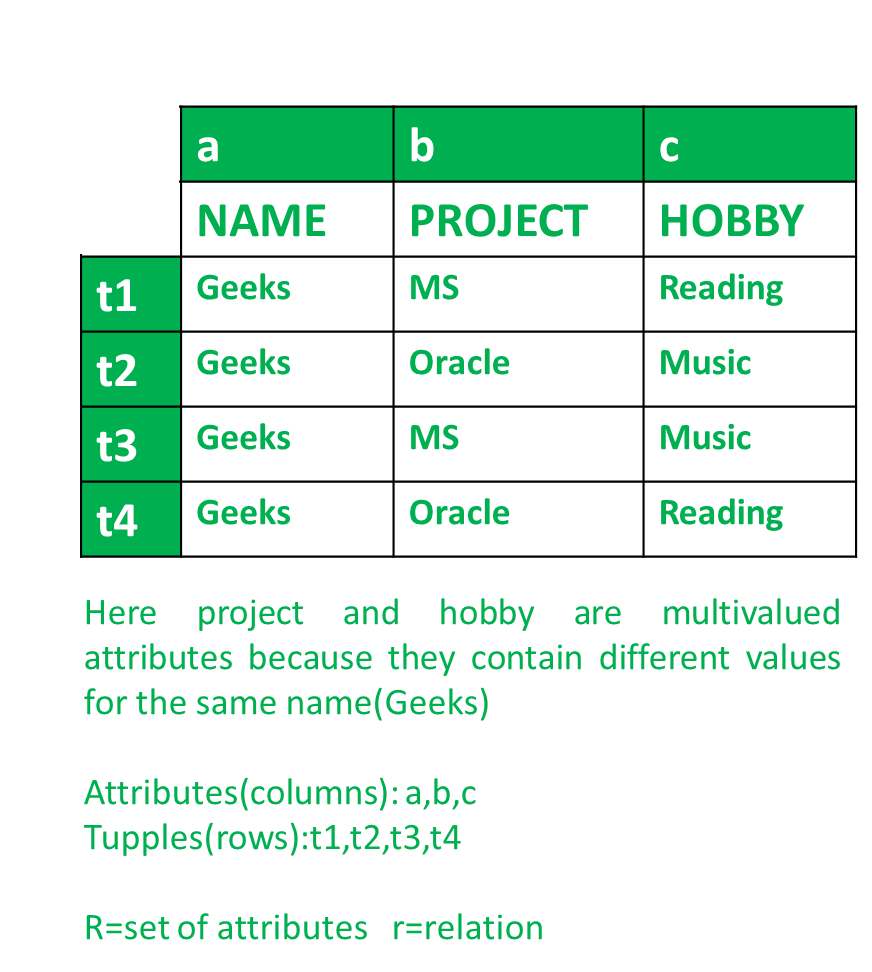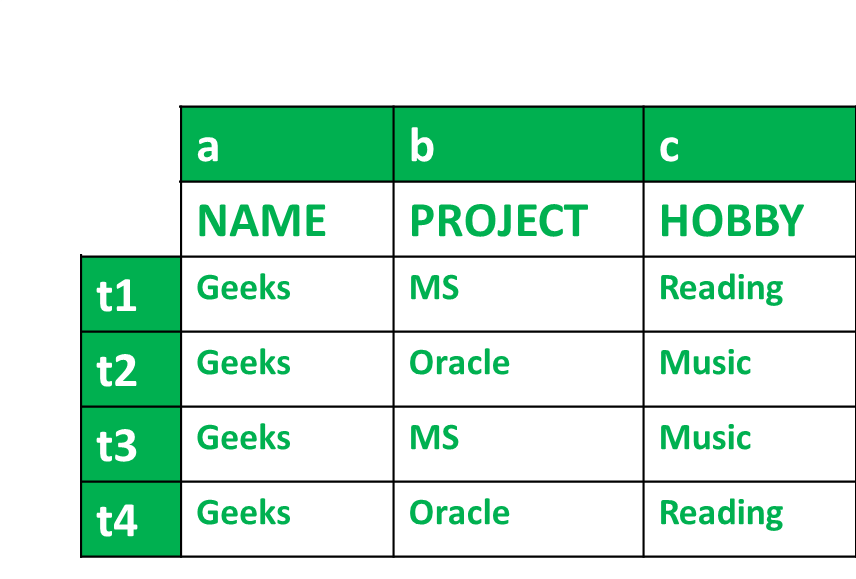# Multivalued Dependency (MVD) in DBMS

• Difficulty Level : Medium
• Last Updated : 23 Feb, 2022

MVD or multivalued dependency means that for a single value of attribute ‘a’ multiple values of attribute ‘b’ exist. We write it as,

`a --> --> b `

It is read as: a is multi-valued dependent on b.

Suppose a person named Geeks is working on 2 projects Microsoft and Oracle and has 2 hobbies namely Reading and Music. This can be expressed in a tabular format in the following way.Project and Hobby are multivalued attributes as they have more than one value for a single person i.e., Geeks.

Multi Valued Dependency (MVD) :
We can say that multivalued dependency exists if the following conditions are met.

Conditions for MVD :
Any attribute say a multiple define another attribute b; if any legal relation r(R), for all pairs of tuples t1 and t2 in r, such that,

`t1[a] = t2[a] `

Then there exists t3 and t4 in r such that.

```t1[a] = t2[a] = t3[a] = t4[a]
t1[b] = t3[b]; t2[b] = t4[b]
t1 = t4; t2 = t3 ```

Then multivalued (MVD) dependency exists.

To check the MVD in given table, we apply the conditions stated above and we check it with the values in the given table.Condition-1 for MVD –

`t1[a] = t2[a] = t3[a] = t4[a] `

Finding from table,

`t1[a] = t2[a] = t3[a] = t4[a] = Geeks `

So, condition 1 is Satisfied.

Condition-2 for MVD –

```t1[b] = t3[b]
And
t2[b] = t4[b] ```

Finding from table,

```t1[b] = t3[b] = MS
And
t2[b] = t4[b] = Oracle ```

So, condition 2 is Satisfied.

Condition-3 for MVD –

```∃c ∈ R-(a ∪ b) where R is the set of attributes in the relational table.
t1 = t4
And
t2=t3 ```

Finding from table,

```t1 = t4 = Reading
And
t2 = t3 = Music ```

So, condition 3 is Satisfied.

All conditions are satisfied, therefore,

`a --> --> b `

According to table we have got,

`name --> --> project `

And for,

`a --> --> C `

We get,

`name --> --> hobby `

Hence, we know that MVD exists in the above table and it can be stated by,

```name --> --> project
name --> --> hobby ```
My Personal Notes arrow_drop_up